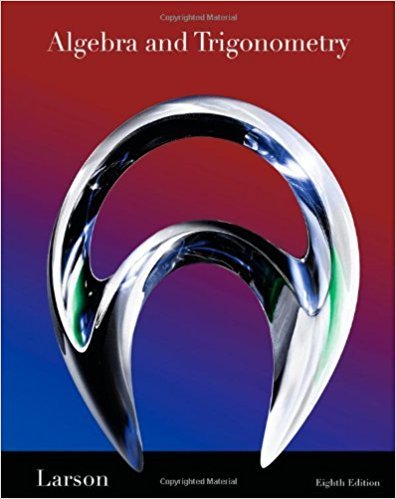×
×

# Solutions for Chapter 11.5: The Binomial Theorem## Full solutions for Algebra and Trigonometry | 8th Edition

ISBN: 9781439048474Solutions for Chapter 11.5: The Binomial Theorem

Solutions for Chapter 11.5
4 5 0 268 Reviews
31
5
##### ISBN: 9781439048474

This textbook survival guide was created for the textbook: Algebra and Trigonometry, edition: 8. Chapter 11.5: The Binomial Theorem includes 108 full step-by-step solutions. Since 108 problems in chapter 11.5: The Binomial Theorem have been answered, more than 52026 students have viewed full step-by-step solutions from this chapter. This expansive textbook survival guide covers the following chapters and their solutions. Algebra and Trigonometry was written by and is associated to the ISBN: 9781439048474.

Key Math Terms and definitions covered in this textbook
• Back substitution.

Upper triangular systems are solved in reverse order Xn to Xl.

• Cholesky factorization

A = CTC = (L.J]))(L.J]))T for positive definite A.

• Commuting matrices AB = BA.

If diagonalizable, they share n eigenvectors.

• Condition number

cond(A) = c(A) = IIAIlIIA-III = amaxlamin. In Ax = b, the relative change Ilox III Ilx II is less than cond(A) times the relative change Ilob III lib II· Condition numbers measure the sensitivity of the output to change in the input.

• Cramer's Rule for Ax = b.

B j has b replacing column j of A; x j = det B j I det A

• Diagonal matrix D.

dij = 0 if i #- j. Block-diagonal: zero outside square blocks Du.

• Fibonacci numbers

0,1,1,2,3,5, ... satisfy Fn = Fn-l + Fn- 2 = (A7 -A~)I()q -A2). Growth rate Al = (1 + .J5) 12 is the largest eigenvalue of the Fibonacci matrix [ } A].

• Free variable Xi.

Column i has no pivot in elimination. We can give the n - r free variables any values, then Ax = b determines the r pivot variables (if solvable!).

• Gram-Schmidt orthogonalization A = QR.

Independent columns in A, orthonormal columns in Q. Each column q j of Q is a combination of the first j columns of A (and conversely, so R is upper triangular). Convention: diag(R) > o.

• Incidence matrix of a directed graph.

The m by n edge-node incidence matrix has a row for each edge (node i to node j), with entries -1 and 1 in columns i and j .

• Indefinite matrix.

A symmetric matrix with eigenvalues of both signs (+ and - ).

• Jordan form 1 = M- 1 AM.

If A has s independent eigenvectors, its "generalized" eigenvector matrix M gives 1 = diag(lt, ... , 1s). The block his Akh +Nk where Nk has 1 's on diagonall. Each block has one eigenvalue Ak and one eigenvector.

• Kronecker product (tensor product) A ® B.

Blocks aij B, eigenvalues Ap(A)Aq(B).

• Krylov subspace Kj(A, b).

The subspace spanned by b, Ab, ... , Aj-Ib. Numerical methods approximate A -I b by x j with residual b - Ax j in this subspace. A good basis for K j requires only multiplication by A at each step.

• lA-II = l/lAI and IATI = IAI.

The big formula for det(A) has a sum of n! terms, the cofactor formula uses determinants of size n - 1, volume of box = I det( A) I.

• Normal matrix.

If N NT = NT N, then N has orthonormal (complex) eigenvectors.

• Orthogonal subspaces.

Every v in V is orthogonal to every w in W.

• Rank r (A)

= number of pivots = dimension of column space = dimension of row space.

• Simplex method for linear programming.

The minimum cost vector x * is found by moving from comer to lower cost comer along the edges of the feasible set (where the constraints Ax = b and x > 0 are satisfied). Minimum cost at a comer!

• Singular Value Decomposition

(SVD) A = U:E VT = (orthogonal) ( diag)( orthogonal) First r columns of U and V are orthonormal bases of C (A) and C (AT), AVi = O'iUi with singular value O'i > O. Last columns are orthonormal bases of nullspaces.

×